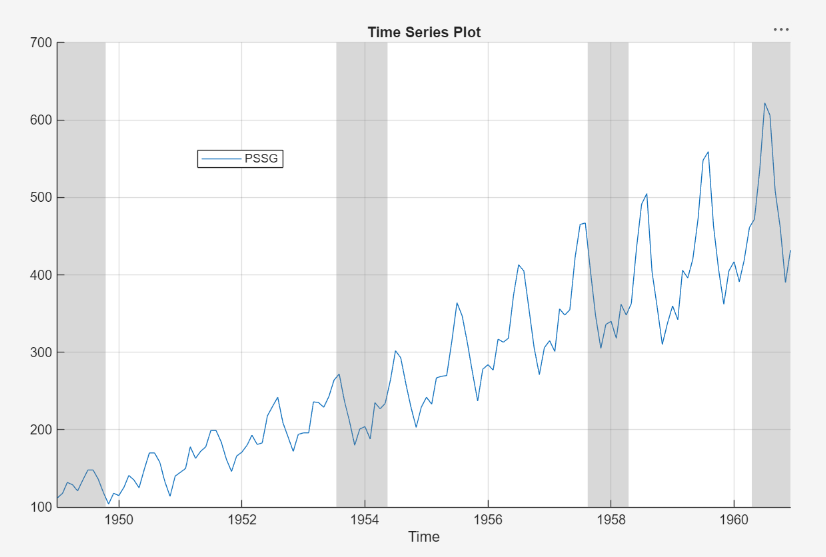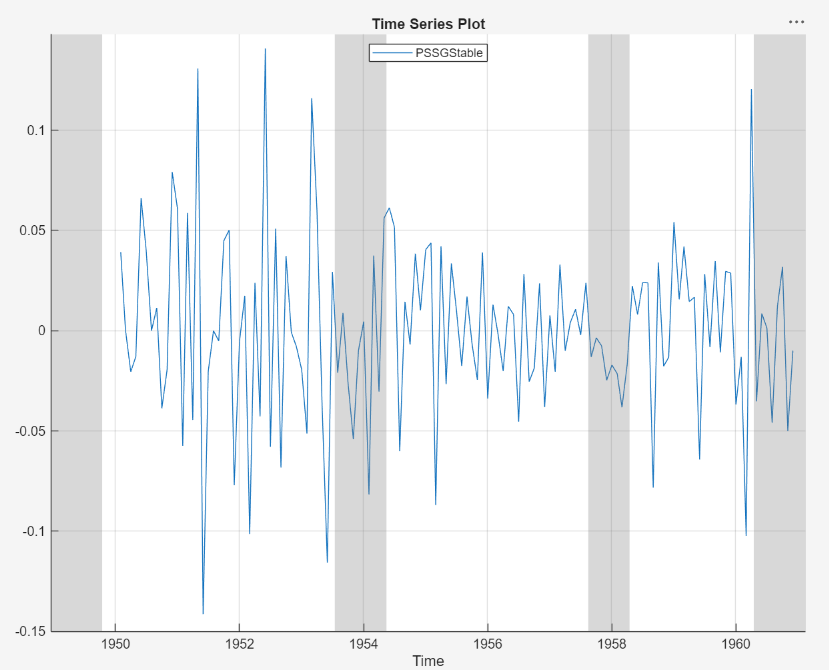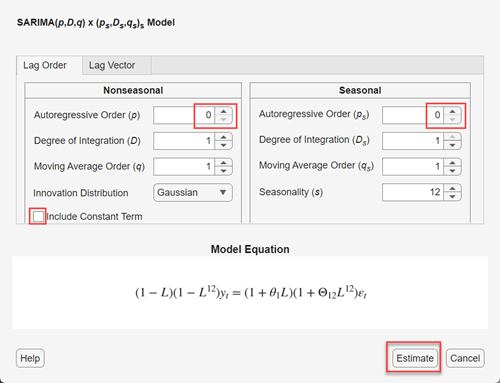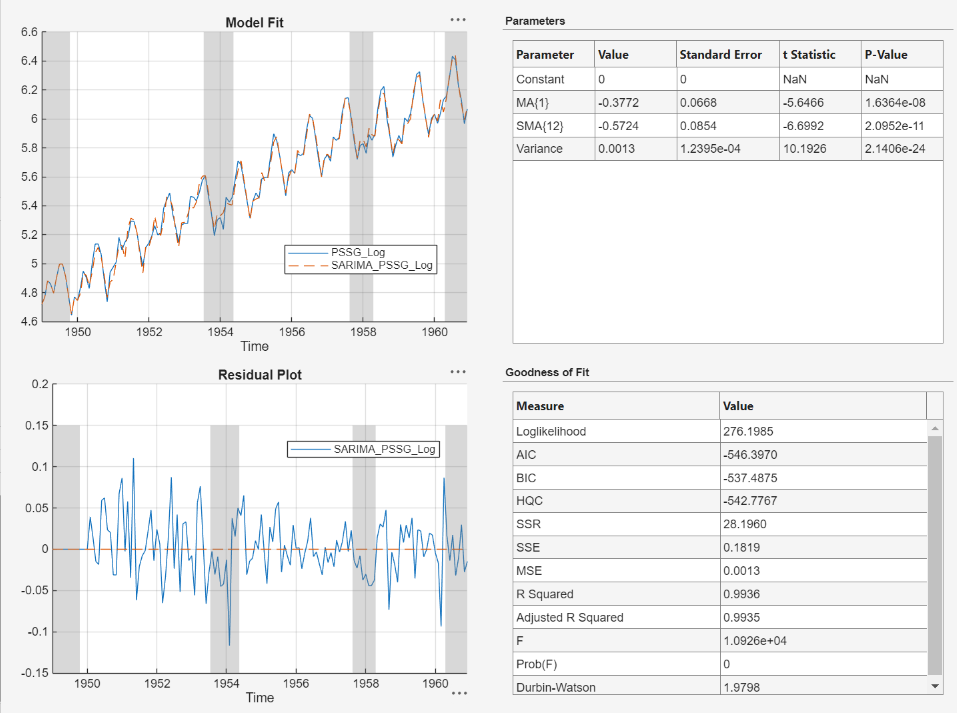Documentation

Estimate Multiplicative ARIMA Model Using Econometric Modeler App

This example shows how to estimate a multiplicative seasonal ARIMA model by using the Econometric Modeler app. The data set, which is stored in mlr/examples/econ/Data_Airline.mat, contains monthly counts of airline passengers. The folder mlr is the value of matlabroot.

Import Data into Econometric Modeler

At the command line, load the Data_Airline.mat data set.

At the command line, open the Econometric Modeler app.

econometricModeler

Alternatively, open the app from the apps gallery (see Econometric Modeler).

Import DataTable into the app:

1. On the Econometric Modeler tab, in the Import section, click.

2. In the Import Data dialog box, in the Import? column, select the check box for the DataTable variable.

3. Click .

The variable PSSG appears in the Data Browser, and its time series plot appears in the Time Series Plot(PSSG) figure window.The series exhibits a seasonal trend, serial correlation, and possible exponential growth. For an interactive analysis of serial correlation, see Detect Serial Correlation Using Econometric Modeler App.

Stabilize Series

Address the exponential trend by applying the log transform to PSSG.

1. In the Data Browser, select PSSG.

2. On the Econometric Modeler tab, in the Transforms section, click .

The transformed variable PSSGLog appears in the Data Browser, and its time series plot appears in the Time Series Plot(PSSGLog) figure window.The exponential growth appears to be removed from the series.

Address the seasonal trend by applying the 12th order seasonal difference. With PSSGLog selected in the Data Browser, on the Econometric Modeler tab, in the Transforms section, set Seasonal to 12. Then, click .

The transformed variable PSSGLogSeasonalDiff appears in the Data Browser, and its time series plot appears in the Time Series Plot(PSSGLogSeasonalDiff) figure window.The transformed series appears to have a unit root.

Test the null hypothesis that PSSGLogSeasonalDiff has a unit root by using the Augmented Dickey-Fuller test. Specify that the alternative is an AR(0) model, then test again specifying an AR(1) model. Adjust the significance level to 0.025 to maintain a total significance level of 0.05.

1. With PSSGLogSeasonalDiff selected in the Data Browser, on the Econometric Modeler tab, in the Tests section, click > Augmented Dickey-Fuller Test.

2. On the ADF tab, in the Parameters section, set Significance Level to 0.025.

3. In the Tests section, click .

4. In the Parameters section, set Number of Lags to 1.

5. In the Tests section, click .

The test results appear in the Results table of the ADF(PSSGLogSeasonalDiff) document.Both tests fail to reject the null hypothesis that the series is a unit root process.

Address the unit root by applying the first difference to PSSGLogSeasonalDiff. With PSSGLogSeasonalDiff selected in the Data Browser, click the Econometric Modeler tab. Then, in the Transforms section, click .

The transformed variable PSSGLogSeasonalDiffDiff appears in the Data Browser, and its time series plot appears in the Time Series Plot(PSSGLogSeasonalDiffDiff) figure window.

Rename the PSSGLogSeasonalDiffDiff variable to PSSGStable:

1. In the Data Browser, right-click PSSGLogSeasonalDiffDiff.

2. In the context menu, select Rename.

3. Enter PSSGStable.

The app updates the names of all documents associated with the transformed series.Identify Model for Series

Determine the lag structure for a conditional mean model of the data by plotting the sample autocorrelation function (ACF) and partial autocorrelation function (PACF).

1. With PSSGStable selected in the Data Browser, click the Plots tab, then click .

2. Show the first 50 lags of the ACF. On the ACF tab, set Number of Lags to 50.

3. Click the Plots tab, then click .

4. Show the first 50 lags of the PACF. On the PACF tab, set Number of Lags to 50.

5. Drag the ACF(PSSGStable) figure window above the PACF(PSSGStable) figure window.According to , the autocorrelations in the ACF and PACF suggest that the following SARIMA(0,1,1)×(0,1,1)12 model is appropriate for PSSGLog.

$\left(1-L\right)\left(1-{L}^{12}\right){y}_{t}=\left(1+{\theta }_{1}L\right)\left(1+{\Theta }_{12}{L}^{12}\right){\epsilon }_{t}.$

Close all figure windows.

Specify and Estimate SARIMA Model

Specify the SARIMA(0,1,1)×(0,1,1)12 model.

1. In the Data Browser, select the PSSGLog time series.

2. On the Econometric Modeler tab, in the Models section, click the arrow > SARIMA.

3. In the SARIMA Model Parameters dialog box, on the Lag Order tab:

• Nonseasonal section

1. Set Degrees of Integration to 1.

2. Set Moving Average Order to 1.

3. Clear the Include Constant Term check box.

• Seasonal section

1. Set Period to 12 to indicate monthly data.

2. Set Moving Average Order to 1.

3. Select the Include Seasonal Difference check box.4. Click .

The model variable SARIMA_PSSGLog appears in the Data Browser, and its estimation summary appears in the Model Summary(SARIMA_PSSGLog) document.The results include:

• Model Fit — A time series plot of PSSGLog and the fitted values from SARIMA_PSSGLog.

• Residual Plot — A time series plot of the residuals of SARIMA_PSSGLog.

• Parameters — A table of estimated parameters of SARIMA_PSSGLog. Because the constant term was held fixed to 0 during estimation, its value and standard error are 0.

• Goodness of Fit — The AIC and BIC fit statistics of SARIMA_PSSGLog.

 Box, G. E. P., G. M. Jenkins, and G. C. Reinsel. Time Series Analysis: Forecasting and Control. 3rd ed. Englewood Cliffs, NJ: Prentice Hall, 1994.